# Lesson 16 Asynchronous GeneratorsInduction Generators ET 332 b

• Slides: 16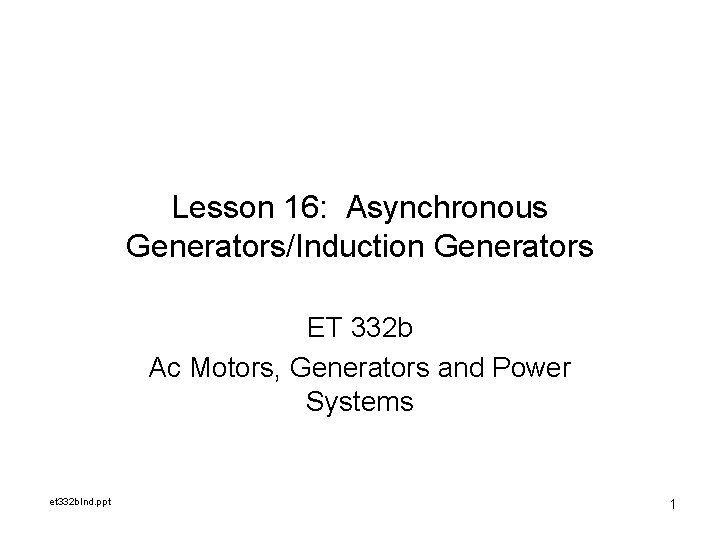Lesson 16: Asynchronous Generators/Induction Generators ET 332 b Ac Motors, Generators and Power Systems et 332 b. Ind. ppt 1Learning Objectives After this presentation you will be able to: Ø Ø Explain how an induction generator operates List application for induction generators in the use renewable resources Discuss the limitations of induction generators Compute the power developed from an induction generator using the per phase circuit model et 332 b. Ind. ppt 2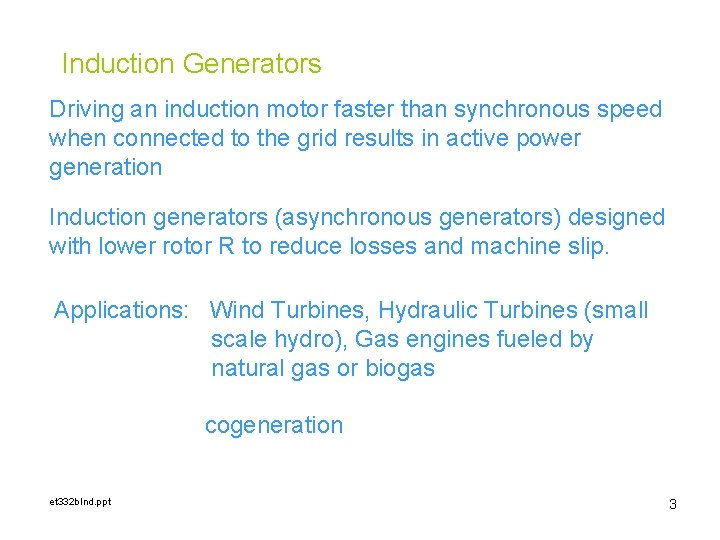Induction Generators Driving an induction motor faster than synchronous speed when connected to the grid results in active power generation Induction generators (asynchronous generators) designed with lower rotor R to reduce losses and machine slip. Applications: Wind Turbines, Hydraulic Turbines (small scale hydro), Gas engines fueled by natural gas or biogas cogeneration et 332 b. Ind. ppt 3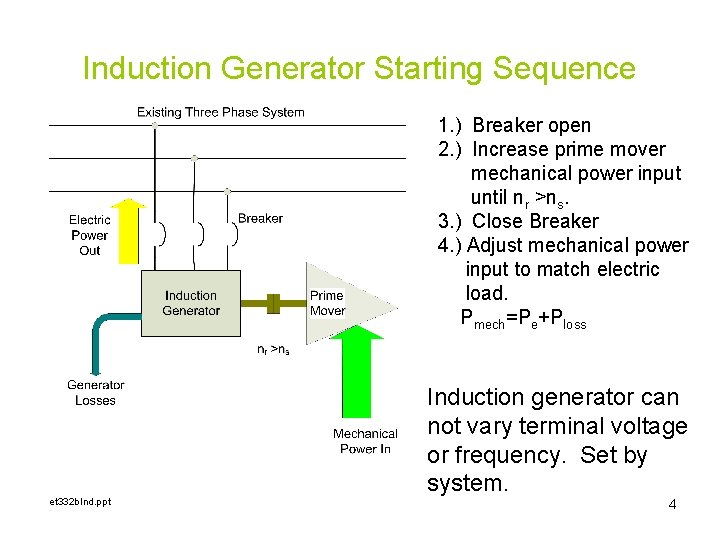Induction Generator Starting Sequence 1. ) Breaker open 2. ) Increase prime mover mechanical power input until nr >ns. 3. ) Close Breaker 4. ) Adjust mechanical power input to match electric load. Pmech=Pe+Ploss et 332 b. Ind. ppt Induction generator can not vary terminal voltage or frequency. Set by system. 4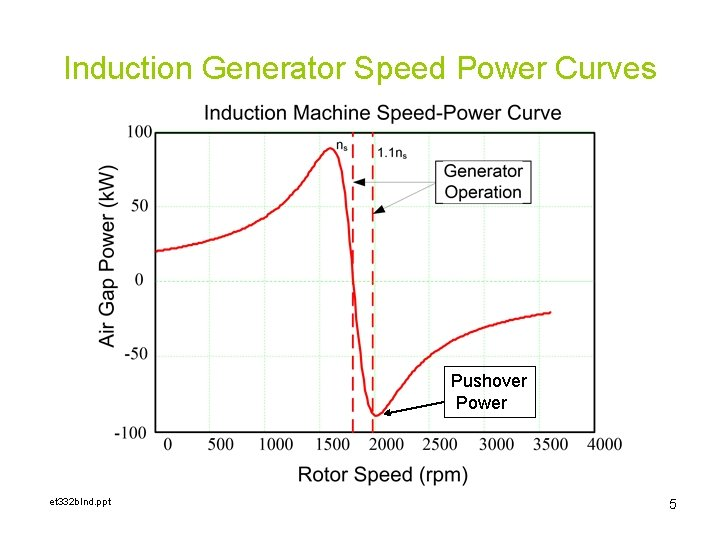Induction Generator Speed Power Curves Pushover Power et 332 b. Ind. ppt 5Limitations of Induction Generations • Require existing power grid for synchronous operation. – Can not control frequency or voltage independently • Can not operate above pushover speed • Require a source of reactive power to operate – When connect to grid, system supplies reactive power to operate generator • When operating without grid connection frequency varies with power output. – Parallel capacitors supply reactive power et 332 b. Ind. ppt 6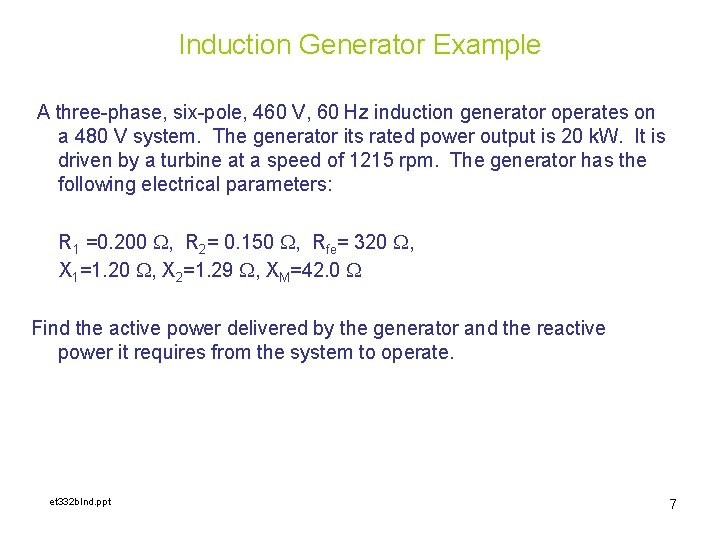Induction Generator Example A three-phase, six-pole, 460 V, 60 Hz induction generator operates on a 480 V system. The generator its rated power output is 20 k. W. It is driven by a turbine at a speed of 1215 rpm. The generator has the following electrical parameters: R 1 =0. 200 W, R 2= 0. 150 W, Rfe= 320 W, X 1=1. 20 W, X 2=1. 29 W, XM=42. 0 W Find the active power delivered by the generator and the reactive power it requires from the system to operate. et 332 b. Ind. ppt 7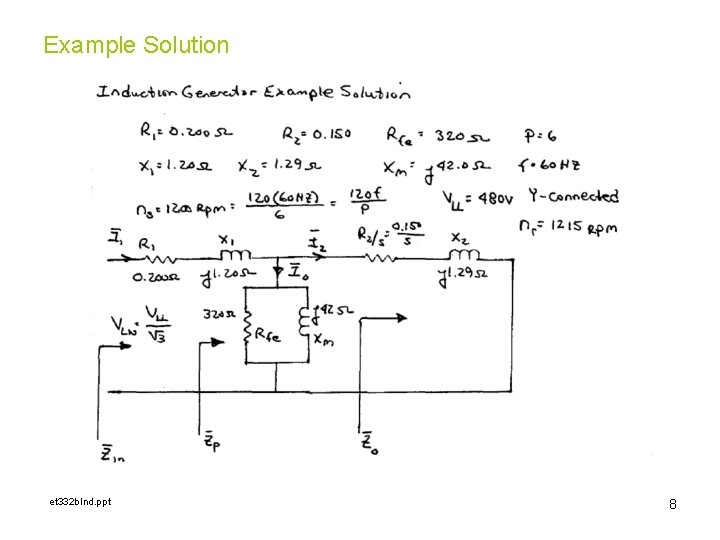Example Solution et 332 b. Ind. ppt 8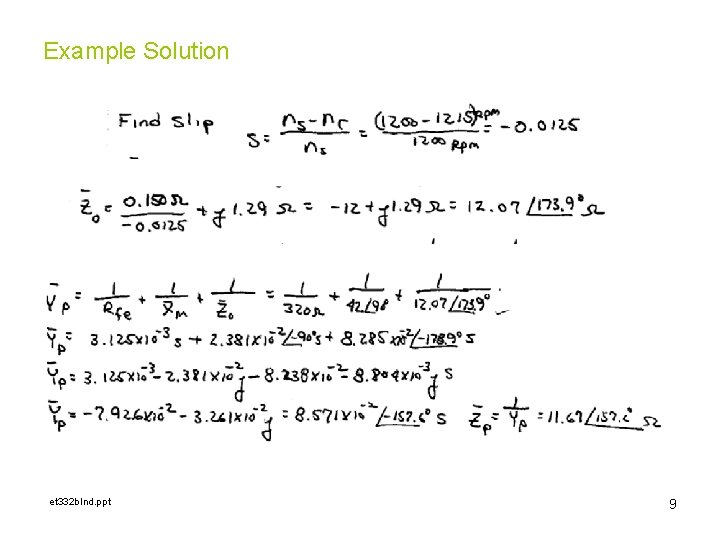Example Solution et 332 b. Ind. ppt 9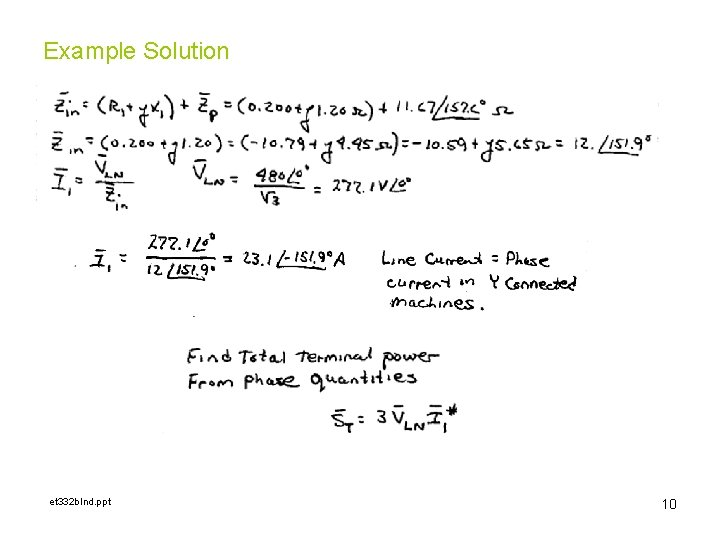Example Solution et 332 b. Ind. ppt 10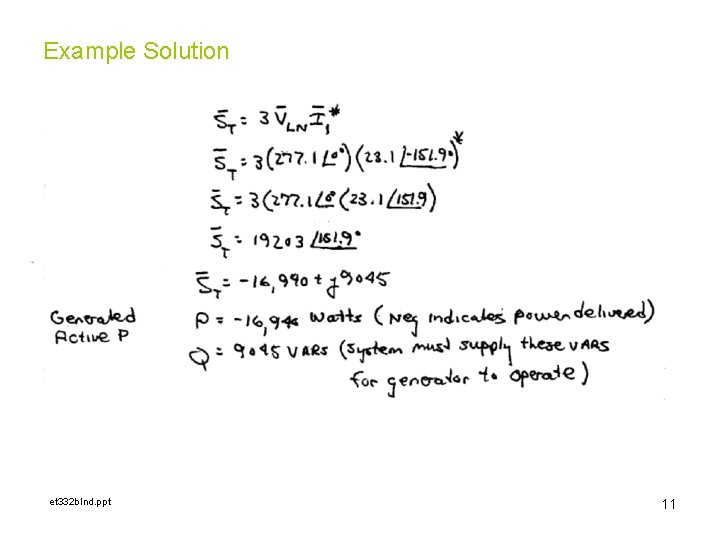Example Solution et 332 b. Ind. ppt 11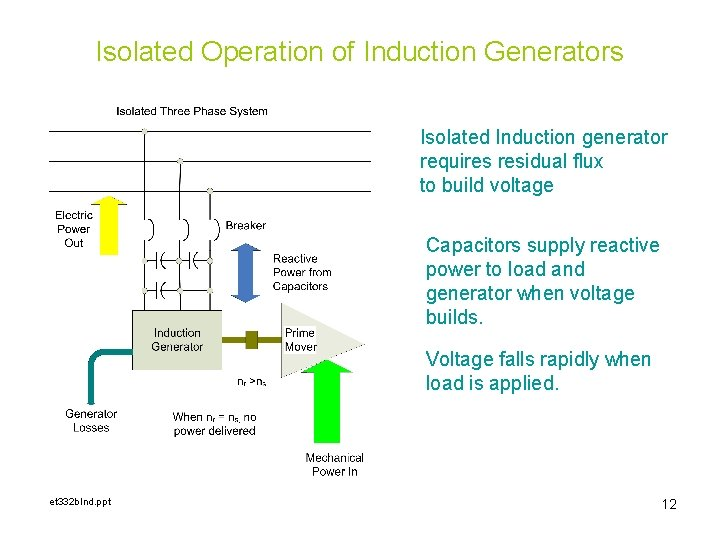Isolated Operation of Induction Generators Isolated Induction generator requires residual flux to build voltage Capacitors supply reactive power to load and generator when voltage builds. Voltage falls rapidly when load is applied. et 332 b. Ind. ppt 12Voltage Build-up in Isolated Induction Generators External Capacitor provides Reactive power for operation Operating point set by intersection between magnetization curve and Xc et 332 b. Ind. ppt 13Voltage Build-up in Isolated Induction Generators Lab measurements determine the magnetization curve Inductance change due to rotor motion et 332 b. Ind. ppt 14Voltage Build-up in Isolated Induction Generators Single phase motor magnetization curve et 332 b. Ind. ppt 15ET 332 b Ac Motors, Generators and Power Systems END LESSON 16: ASYNCHRONOUS GENERATORS/INDUCTION GENERATORS et 332 b. Ind. ppt 16